# Sequential probability ratio test

SPRT

Letbe a sequence of independent random variables with common discrete or continuous probability density function(cf. also Random variable; Distribution function). In testing the hypotheses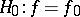versus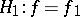(cf. also Statistical hypotheses, verification of), the probability ratio or likelihood ratio afterobservations is given by (cf. also Likelihood-ratio test)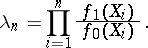(a1)

In the Neyman–Pearson probability ratio test, one fixes a sample size, chooses a valueand decides to accept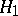if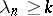and decides to acceptif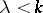. In the sequential probability ratio test introduced by A. Wald [a5], the sample size is not chosen ahead of time. Instead, one chooses two positive constantsand sequentially computes the likelihood ratio after each observation. If afterobservations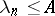, one stops taking observations and decides to accept. If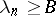, one stops and decides to accept. Otherwise, another observation is taken. The number of observations taken is thus a random variable, which can be shown to be finite with probability one.

Denote the error probabilities of this procedure by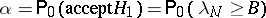and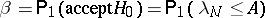. It then follows that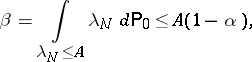(a2)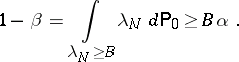If the likelihood ratio always hits the boundary when the test stops, so that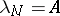or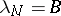, then these inequalities become equalities. Otherwise, the inequalities become close approximations in the standard cases.

The logarithm of the likelihood ratio as given in (a1) is a sum of independent, identically distributed random variables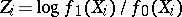. It then follows from Wald's lemma that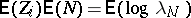. Using the same type of approximations as above, this gives the following formulas for the average sample number of the test: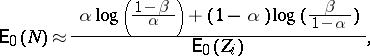(a3)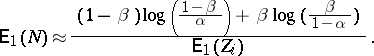If the likelihood ratio always hits the boundary when the test stops, these approximations become equalities.

Wald and J. Wolfowitz [a6] proved a strong optimality property for the sequential probability ratio test. It states that among all sequential tests with error probabilities no bigger than that of a given sequential probability ratio test, the sequential probability ratio test has the smallest average sample number under both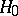and. Indeed, the average savings in sampling relative to the Neyman–Pearson test with the same error probabilities is 50% or more in many cases (see [a2] for details).

In most realistic situations the hypotheses to be tested are composite, the probability distributions are parametrized by some parameter, and one is interested in testing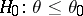versus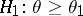. In such a case one can perform the sequential probability ratio test for the simple hypotheses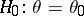versus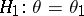. In the most important cases one can apply the fundamental identity of sequential analysis (see [a2] for details) to find the approximate power functions and average sample number functions for such procedures. However, even when such tests achieve specified error probabilities for all values of the parameter, the Wald–Wolfowitz optimality will not carry over to values ofother thanand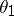. Indeed, the expected sample size may even exceed that of the corresponding fixed sample size test at some values ofbetween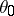and.

It is because of this phenomenon that J. Kiefer and L. Weiss [a4] raised the question of finding the sequential test with given error probabilities atand, which minimizes the maximum expected sample size over all. To solve this problem, G. Lorden [a3] introduced a test based on performing two one-sided sequential probability ratio tests simultaneously. First, a third value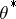is chosen. Test one is then a sequential probability ratio test ofversus, where the constant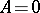. Test two is a sequential probability ratio test of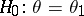versus, where. The-sequential probability ratio test stops and makes its decision as soon as either one of the individual sequential probability ratio tests stops. The decision is to acceptif test one stops first and the decision is to acceptif the second test stops first. It can be shown that for the proper choice ofthis test does asymptotically solve the Kiefer–Weiss problem (see [a1] for a simple way to select).

The sequential probability ratio test can also be applied in situations where the observations are not independent and identically distributed. In such a situation the likelihood ratio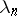can be more difficult to compute at each stage. The inequalities (a2) continue to hold for such a sequential probability ratio test, but the formulas (a3) for the average sample number are no longer valid. The sequential probability ratio test has also been studied where the observations form a continuous-time stochastic process. In fact, parts of the theory simplify in such a situation, since the likelihood ratio process often becomes a process with continuous sample paths and thus always hits the boundary when it stops.

How to Cite This Entry:
Sequential probability ratio test. Bennett Eisenberg (originator), Encyclopedia of Mathematics. URL: http://encyclopediaofmath.org/index.php?title=Sequential_probability_ratio_test&oldid=12721
This text originally appeared in Encyclopedia of Mathematics - ISBN 1402006098